# Word Embedding of Brown Corpus Using Python

For this project, the basic idea is words that tend to appear in similar context are likely to be related. You shall know a word by the company it keeps (Firth, 1957). Standing on this concept, this project is mainly investigated an embedding of words that is based on co-occurrence statistics.

Data source: Brown corpus is a collection of text samples from a wide range of sources, with a total of over a million words. The analysis of this project is mainly based on Brown corpus.

Download brown corpus data from nltk.corpus (brown.words).

`import nltkimport matplotlib as pltimport pandas as pdimport mathfrom nltk.corpus import brownimport numpy as npnltk.download('brown')brown.words()[:10]['The', 'Fulton', 'County', 'Grand', 'Jury', 'said', 'Friday', 'an', 'investigation', 'of']`
1. Import the stop words from nltk.corpus, meanwhile import the punctuation from string. However, the punctuation of string missed some punctuation. Combining the string and extra punctuations appeared in brown.words, I create a new punctuations list (punctuation).

# Remove stopwords and punctuation,lowercase every word, and count word frequncy

`from nltk.corpus import stopwordsnltk.download('stopwords')import string# I found that string.punctuation did not include all the punctuations of brown words, here I added extra punctuation in the following listpunctuation = ['!','"','#','\$','%','&',"'",'(',')','*','+',',','-','.','/',':',';','<','=','>','?','@','[','\\',']','^','_','`','{','|','}','~','``',"''",'--']lower_words = [x.lower() for x in brown.words()]pun_stop = punctuation + stopwords.words('english')filter_words1 = [x for x in lower_words if x not in pun_stop]filter_words = list(filter(lambda x: x.isalpha() and len(x) > 1, filter_words1)) # remove numbers and single letter words`

# Extract the first 5000 and first 1000 most commonly-occurring words

1. Count each word frequency in filtered data, and form a word-frequency dictionary (words_count).
`import collectionsimport operatorwords_count = dict(collections.Counter(filter_words))sorted_words = sorted(words_count.items(), key = operator.itemgetter(1), reverse = True)# first 5000 most commonly-occuring wordsV = [x for x in sorted_words[:5000]]C = V[:1000]`
1. For each target word of V, find the positions of each occurrence of it in filtered data (positions); for each position forming a window (the window size include 5 words, target word in middle, two words before and two words after: [index-2:index]+filter_words[index+1:index+3] and index in positions), look at the surrounding four words, and count how often these surrounding words appear in context word list C (cword_fre).
`import itertoolsdef ls_uniq(seq):    checked = []   for e in seq:       if e not in checked:           checked.append(e)   return checkedc_words = []for v_word in V:    four_words = []    positions = [x for x, n in enumerate(filter_words) if n == v_word] # locate each word of V in filter_words    for i in positions:        if i ==0:            four_word = filter_words[1:3]        elif i == 1:            four_word = ([filter_words] + filter_words[2:4])        else:            four_word = (filter_words[(i-2):i] + filter_words[(i+1):(i+3)])        four_word_uniq = ls_uniq(four_word)        four_words = four_words + four_word_uniq    four_words_count = dict(collections.Counter(four_words))    window_count = len(positions)    for c_word in four_words_count:        if c_word in C:            cword_fre = four_words_count[c_word]            Pr_cw = cword_fre/window_count            c_words.append((v_word, c_word, cword_fre, window_count, Pr_cw))cwords = pd.DataFrame(c_words)cwords.columns = ['V_Word','C_Word','Cword_Count','Window_Count','Pr_cw']cwords.head()`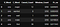Head of Condition Probability and Word Frequency DataFrame

Meanwhile, for each context word of C, count the occurrence in filtered_words list (Count_c), and construct the overall probability distribution in preprocessed data set Pr(c) (Count_c / n, n is the length of preprocessed word list (filter_words)). Combine context words (Cont_words), context words frequency (Cont_counts), and the overall probability of context word (Pr_c) into one data frame Context_words.

`cwords_uniq = list(cwords['C_Word'].unique())cwords_pro = {}for cword in cwords_uniq:    cwords_pro[cword] = filter_words.count(cword) / len(filter_words)def cword_pro(x):    return cwords_pro[x]cwords['Pr_c'] = cwords['C_Word'].apply(cword_pro)cwords.head()`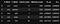Add One Column of Context Words Probability

According to the positive point wise mutual information to represent each target word (w) by context-word dimensional vector(f_w).

Combine all the necessary variables into one table (cwords). These variables include target word (Word), context word (Cword), context word frequency (Cwordfre), window number for each target word (Winum), conditional Probabilty of context word appeared when target word occurs (Pr_cw), overall probability of context word in preprocessed data (Pr_c), and mutual information (f_w).

`def max_log(row):    f = row['Pr_cw']    g = row['Pr_c']    l = math.log(f/g)    return max(0, l)cwords['f_w'] = cwords.apply(max_log, axis = 1)cwords.head()`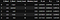Build Final Data Frame

In order to reduce matrix dimension, using pd.pivot_table stack Word (target word), Cword (context word), and f_w (mutual information) from cwords dataframe to construct a new data frame (mutal_words). For the new table, Word as index, Cwords as columns, and f_w as value. However, the new table is pretty sparse and includes many NaN values. In order for the following analysis, fill all the NaN values with 0.

`mutal_words = pd.pivot_table(cwords, index = 'V_Word', columns = 'C_Word', values = 'f_w')mutal_words.head()`
`mutal_words = mutal_words.fillna(0)mutal_words.head()`

This step is about the dimension reduction. Features are Cword (1000). The above table shows us that our data are very sparse. When we do dimension reduction, for high dimensional data, generally PCA is used to decompose the data. However, for the high dimensional sparse text data set (like our data set), singular Value Decomposition (SVD) is better. Therefore, in this project we use TruncatedSVD to decompose our data. Finally we get the 100-dimension-word representation.

`from sklearn.decomposition import TruncatedSVDfrom sklearn.random_projection import sparse_random_matrixX = np.asarray(mutal_words)svd = TruncatedSVD(n_components = 100, n_iter = 7, random_state = 42)svd.fit(X)X_reduce = svd.fit_transform(X)X_reduce_df = pd.DataFrame(X_reduce, index = mutal_words.index)X_reduce_df.head()`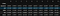Dimension Reduction with TruncatedSVD

Use package of cosine_similarity of sklearn.metrics.pairwise to calculate the cosine distance of each pair of target word, and get the symmetrical matrix (dist). Transform this matrix into a data frame (dist_df). For this table, each row represents the distance of one target word to all the other target word.

The purpose of this step is to find the nearest neighbor of picked word. Nearest neighbor means the minimum distance and maximum similarity. However, for each word, the distance to itself is minimum (close to zero, in diagonal of matrix). In order to find other nearest neighbor (not the word itself), set the value of diagonal to 1.

`from sklearn.metrics.pairwise import cosine_similarity as cswords_similarity = 1 - cs(X_reduce, X_reduce)words_similarity_df = pd.DataFrame(words_similarity, index = mutal_words.index,columns = mutal_words.index)np.fill_diagonal(words_similarity_df.values, 1)words_similarity_df.head()`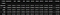The similarity DataFrame

Randomly pick up 16 meaningful words from V word list

word_list = [‘room’, ‘community’, ‘america’, ‘washington’, ‘nature’, ‘europe’, ‘hospital’, ‘cities’, ‘leaders’, ‘communist’, ‘chicago’, ‘conference’, ‘ideas’, ‘production’, ‘black’, ‘north’]

Use cosine distance to measure the distance, find the nearest neighbor of each word, finally the nearest neighbor of each word is:

`# according to similarity matrix to find the cloest meaning wordwords_list = ['room', 'community', 'america', 'washington','nature', 'europe', 'hospital', 'cities', 'leaders', 'communist', 'chicago', 'conference', 'ideas', 'production', 'black', 'north']words_similarity_dict = {}for word_list in words_list:    words_similarity_dict[word_list] = words_similarity_df[word_list].idxmin()for word_dict in words_similarity_dict:    print ('{} ------> {}'.format(word_dict, words_similarity_dict[word_dict]))`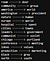Closest Words Pairs

This post explained the whole word embedding procedures. Word embedding will map each word in a function approximation problem into Euclidean spaces, and mapping similar word close to each other in the embedding space it reveals the intrinsic properties of the words. If you have any question please leave your precious comments below, and if you like it, please give a clap.

Data Scientist Costar

## More from Xia Song

Data Scientist Costar